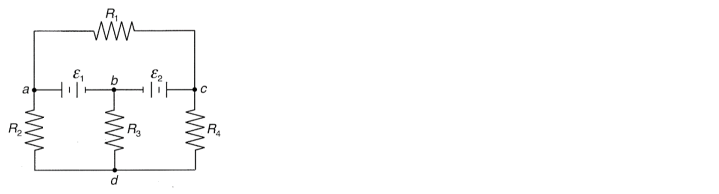# Problem: For the circuit below, determine all the currents. Assume ε1 = 10 V, ε2 = 15 V, R 1 = R 3 = 40 Ω, R 2 = 60 Ω, R 4 = 100 Ω.

🤓 Based on our data, we think this question is relevant for Professor Opeil's class at BC.

###### Problem Details

For the circuit below, determine all the currents. Assume ε1 = 10 V, ε2 = 15 V, R = R = 40 Ω, R 2 = 60 Ω, R 4 = 100 Ω.